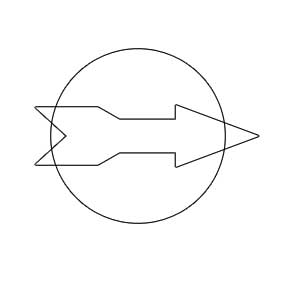SEARCH HOMEMath Central Quandaries & QueriesXetro, a student: Hi, Suppose you have an irregular polygon(convex or concave) with n > 3 sides. The question is - Find some circle that will cut(in limiting case - touch) all the sides of that polygon. It doesn't matter how many times it cuts the side(1 or 2), it just have to cut or touch it. How to find such a circle? or how to decide if such circle even exists? What if those segments do not form a polygon but are some arbitrary segments ? Really want to know how to do it................ Thanks a lot.. Regards, XetroAll irregular polygons do not have a circle that is tangent to each side, the exception is the triangle. If you were to draw a polygon at random, it is unlikely that there is a circle that has every side as a tangent. Irregular polygons do not have a centre but rather a centroid which is the arithmetic mean of each of the coordinate points. For a convex irregular polygon, the centroid lies within the polygon and for a non-convex polygon the centroid lies outside the polygon. If you find the centroid of a irregular, this would be your best bet for finding a circle that cuts all sides of a irregular. Even though it is slightly more likely that you could find a circle that can cut or be tangent to the sides a an irregular polygon, there are still polygons that it would not be possible for a circle to touch all sides. Consider the polygon below:There is no size or position I could put a circle that would touch all sides of the polygon. You can however start with the circle and draw the irregular polygon around it.

Hope this helps,

JaniceMath Central is supported by the University of Regina and The Pacific Institute for the Mathematical Sciences.